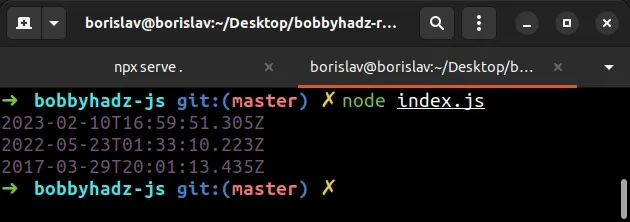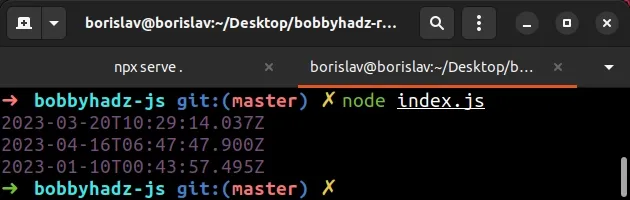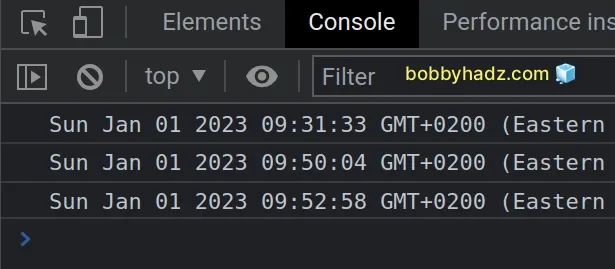# How to generate a random Date in JavaScriptLast updated: Apr 19, 2023
4 min## #How to generate a random Date in JavaScript

To generate a random date in JavaScript:

1. Use the `getTime()` method to get the timestamp of the `from` and `to` dates.
2. Use the `Math.random()` method to generate a random number.
3. Use the `Date()` constructor to convert the summation of the `from` timestamp and the random number.
index.js
```Copied!```function generateRandomDate(from, to) {
return new Date(
from.getTime() +
Math.random() * (to.getTime() - from.getTime()),
);
}

console.log(
generateRandomDate(new Date(2023, 0, 1), new Date()),
);
console.log(
generateRandomDate(new Date(2000, 3, 20), new Date()),
);
console.log(
generateRandomDate(new Date(2010, 5, 25), new Date()),
);
``````The `generateRandomDate` function takes the 2 date objects as parameters:

1. `from` - the min date.
2. `to` - the max date.

The function generates a `Date` object in the given range.

The getTime method returns the number of milliseconds elapsed between the 1st of January 1970 and the given date.

index.js
```Copied!```// 👇️ 1681890643857
console.log(new Date().getTime());
``````

The Math.random() function returns a random number in the range `0` to less than `1`.

The function could return `0` but it could never return `1`.

index.js
```Copied!```console.log(Math.random()); // 👉️ 0.05015971277107356
console.log(Math.random()); // 👉️ 0.2760304811727079
console.log(Math.random()); // 👉️ 0.8000876338556213
``````

We used the subtraction operator to calculate the number of milliseconds between the max and min dates in the range.

index.js
```Copied!```function generateRandomDate(from, to) {
return new Date(
from.getTime() +
Math.random() * (to.getTime() - from.getTime()),
);
}
``````

Here is an example of calculating the difference in milliseconds between the dates.

index.js
```Copied!```const max = new Date();
const min = new Date(2023, 0, 1);

// 👇️ 9366812436
console.log(max.getTime() - min.getTime());
``````

We then multiplied the result by the output of the `Math.random()` method.

The last step is to add the output of calling the `getTime()` method on the end date.

This way, we can be sure that the generated date will be in the specified range.

The `generateRandomDate` function expects to get called with 2 date objects.

index.js
```Copied!```function generateRandomDate(from, to) {
return new Date(
from.getTime() +
Math.random() * (to.getTime() - from.getTime()),
);
}

console.log(
generateRandomDate(new Date(2023, 0, 1), new Date()),
);
console.log(
generateRandomDate(new Date(2000, 3, 20), new Date()),
);
console.log(
generateRandomDate(new Date(2010, 5, 25), new Date()),
);
``````

When using the Date() constructor, keep in mind that it takes a zero-based month as a parameter.

The parameters the constructor takes are:

• year
• month - January = 0, February = 1, March = 2, etc.
• day of the month
• hour
• minutes
• seconds

In other words, if you pass a month of `0` as the second argument to the `Date()` constructor class, then the month of the date is in January.

If you call the `Date()` constructor without passing it any parameters, a Date object that represents the current date and time is created.

index.js
```Copied!```// 👇️ 2023-04-19T08:01:49.947Z
console.log(new Date());
``````

The calls to the `generateRandomDate()` functions in the examples above use the current date and time as the max date in the range.

## #Formatting the randomly generated dates

You can use built-in methods such as toLocaleString and toLocaleDateString() if you need to format the randomly generates dates.

index.js
```Copied!```function generateRandomDate(from, to) {
return new Date(
from.getTime() +
Math.random() * (to.getTime() - from.getTime()),
);
}

console.log(
generateRandomDate(
new Date(2023, 0, 1),
new Date(),
).toLocaleDateString(),
);
console.log(
generateRandomDate(
new Date(2000, 3, 20),
new Date(),
).toLocaleString(),
);
``````If you need to format the randomly generate date in a different way, check out my other articles:

## #Setting default parameters for the start and end dates

In some cases, you might have a predefined range in which the randomly generated dates should fall.

If that's the case, use default parameters, so the function can be invoked without supplying any arguments.

index.js
```Copied!```function generateRandomDate(
from = new Date(2023, 0, 1),
to = new Date(),
) {
return new Date(
from.getTime() +
Math.random() * (to.getTime() - from.getTime()),
);
}

console.log(generateRandomDate());
console.log(generateRandomDate());
console.log(generateRandomDate());
``````We set the `from` date to the first of January 2023 and the `to` date to the current date.

## #Specifying the start and end times when generating the time

You can also explicitly set the start and end times of the randomly generated date.

index.js
```Copied!```function generateRandomDate(from, to) {
return new Date(
from.getTime() +
Math.random() * (to.getTime() - from.getTime()),
);
}

console.log(
generateRandomDate(
new Date(2023, 0, 1, 9, 30, 30),
new Date(2023, 0, 1, 10),
),
);

console.log(
generateRandomDate(
new Date(2023, 0, 1, 9, 30, 30),
new Date(2023, 0, 1, 10),
),
);

console.log(
generateRandomDate(
new Date(2023, 0, 1, 9, 30, 30),
new Date(2023, 0, 1, 10),
),
);
``````

The calls to the `generateRandomDate()` function use the first of January as the start and end date in the range.

The start time is 09:30:30 AM and the stop time is 10:00:00.As shown in the screenshot, all of the generated Dates are in the given range.